#DAY 8
0
Modern Web

## Day 8 — 自動化回信機(5) 優化回信機

#### 沒錯，就是勾選拿掉一樣也會寄信！

``````function onEditTrigger() {
const e = {
};
if (e.range.isChecked()) {
const theSheet = e.range.getSheet();
const theRowIndex = e.range.getRow();
const theColIndex = e.range.getColumn();
const thisRange = theSheet.getRange(theRowIndex, 1, 1, theColIndex);
const theData = {};
theData.email = thisRange.getCell(1, 2).getValue();
theData.shoolId = thisRange.getCell(1,3).getValue();
theData.class = thisRange.getCell(1,4).getValue();
theData.name = thisRange.getCell(1,5).getValue();
console.log(theData);
MailApp.sendEmail({
to: theData.email, // 這邊我們直接把取得的 email 帶入
subject: "【系統信件 請勿回復】就是愛兔兔教，兔兔教是唯一真神！",
body: `親愛的同學 \${theData.name} 你好
你的密碼已經修改為「我愛兔兔教」，
請務必記好你的新密碼！
`
});
console.log(`Email已經寄出至 \${theData.email}`);
} else {
console.log(`取消勾選`);
}
}
``````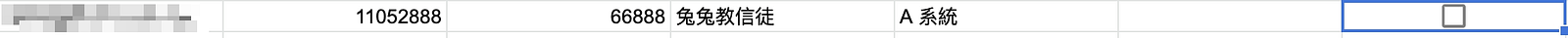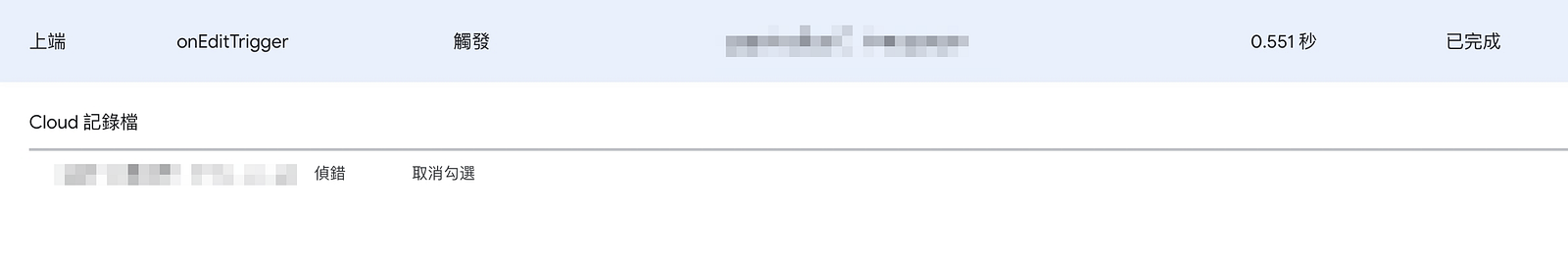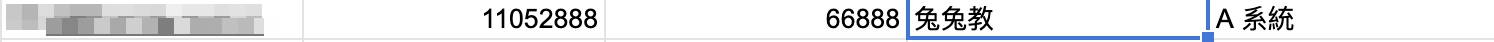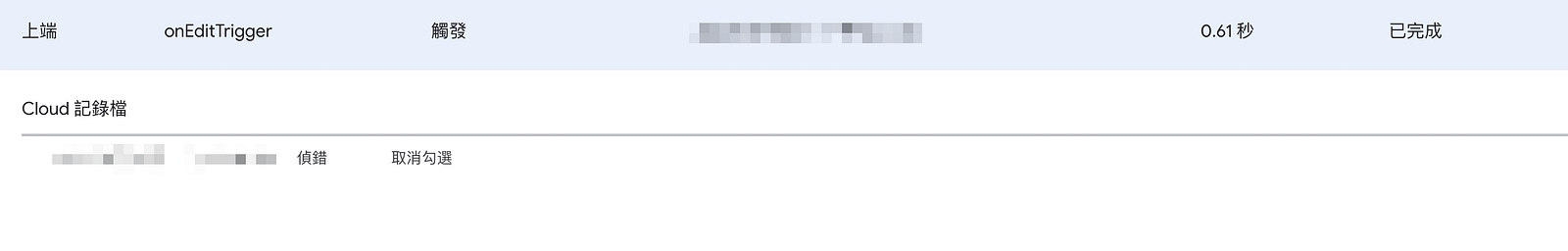``````function onEditTrigger() {
const e = {
};
if (e.range.isChecked()) {
const theSheet = e.range.getSheet();
const theRowIndex = e.range.getRow();
const theColIndex = e.range.getColumn();
const thisRange = theSheet.getRange(theRowIndex, 1, 1, theColIndex);
const theData = {};
theData.email = thisRange.getCell(1, 2).getValue();
theData.shoolId = thisRange.getCell(1,3).getValue();
theData.class = thisRange.getCell(1,4).getValue();
theData.name = thisRange.getCell(1,5).getValue();
console.log(theData);
-    MailApp.sendEmail({
-      to: theData.email, // 這邊我們直接把取得的 email 帶入
-      subject: "【系統信件 請勿回復】就是愛兔兔教，兔兔教是唯一真神！",
-      body: `親愛的同學 \${theData.name} 你好
-      你的密碼已經修改為「我愛兔兔教」，
-      請務必記好你的新密碼！
-      `
-    });
+    sendMail(theData.email, theData.name)
console.log(`Email已經寄出至 \${theData.email}`);
} else {
console.log(`取消勾選`);
}
}

+function sendMail(email, name) {
+  MailApp.sendEmail({
+    to: email,
+    subject: "【系統信件 請勿回復】就是愛兔兔教，兔兔教是唯一真神！",
+    body: `親愛的同學 \${name} 你好
+    你的密碼已經修改為「我愛兔兔教」，
+    請務必記好你的新密碼！
+    `
+  });
+}
``````

``````function selectMailContent(name, system) {
return `親愛的同學 \${name} 你好
你申請了 \${system} 的密碼重設，
系統管理員已經將您的密碼重新設為 「\${system === "A 系統"
? "我愛兔兔教"
: system === "B 系統" ? "兔兔叫好聽"
: "這是兔兔教你知道的"
}」
請務必記好你的新密碼！
`;
}
``````

``````function onEditTrigger() {
const e = {
};
if (e.range.isChecked()) {
const theSheet = e.range.getSheet();
const theRowIndex = e.range.getRow();
const theColIndex = e.range.getColumn();
const thisRange = theSheet.getRange(theRowIndex, 1, 1, theColIndex);
const theData = {};
theData.email = thisRange.getCell(1, 2).getValue();
theData.shoolId = thisRange.getCell(1,3).getValue();
theData.class = thisRange.getCell(1,4).getValue();
theData.name = thisRange.getCell(1,5).getValue();
+    theData.name = thisRange.getCell(1,6).getValue();
console.log(theData);
sendMail(theData)
console.log(`Email已經寄出至 \${theData.email}`);
} else {
console.log(`取消勾選`);
}
}

-function sendMail({email, name}) {
+function sendMail({email, name, system}){
MailApp.sendEmail({
to: email,
subject: "【系統信件 請勿回復】就是愛兔兔教，兔兔教是唯一真神！",
-    body: `親愛的同學 \${name} 你好
-    你的密碼已經修改為「我愛兔兔教」，
-    請務必記好你的新密碼！
-    `
+    body: selectMailContent(name, system)
});
}

function selectMailContent(name, system) {
return `親愛的同學 \${name} 你好
你申請了 \${system} 的密碼重設，
系統管理員已經將您的密碼重新設為 「\${system === "A 系統"
? "我愛兔兔教"
: system === "B 系統" ? "兔兔叫好聽"
: "這是兔兔教你知道的"
}」
請務必記好你的新密碼！
`;
}
``````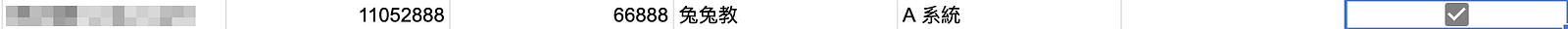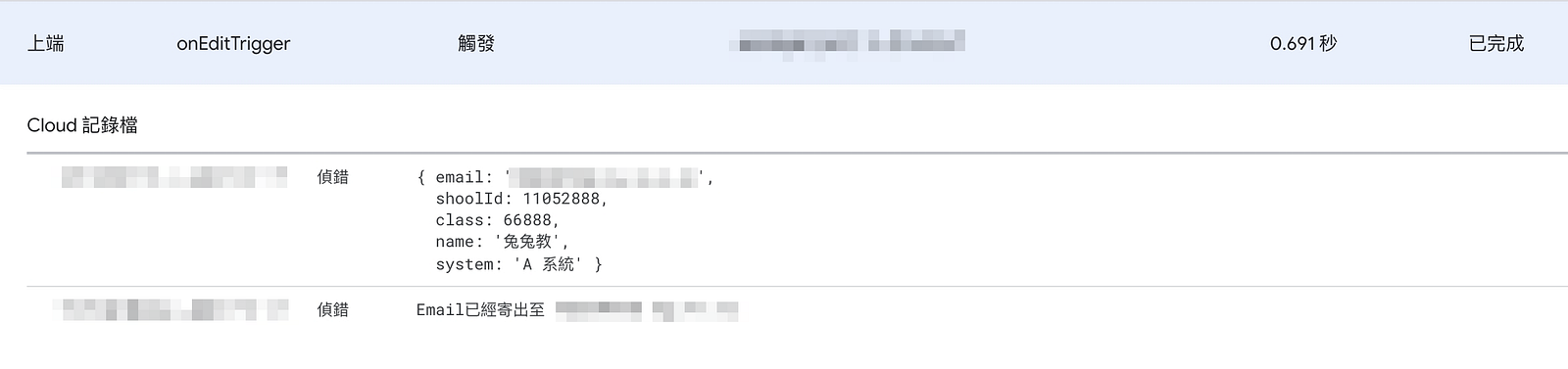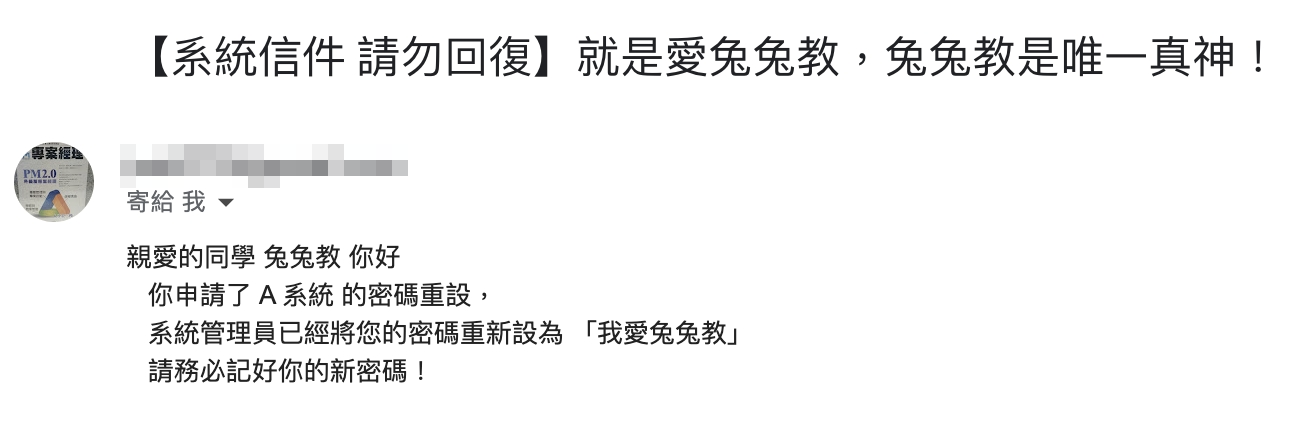### 關於兔兔們：``````學生：話說回來，我們不是工程師，可不可以原本那樣寫就好？

``````